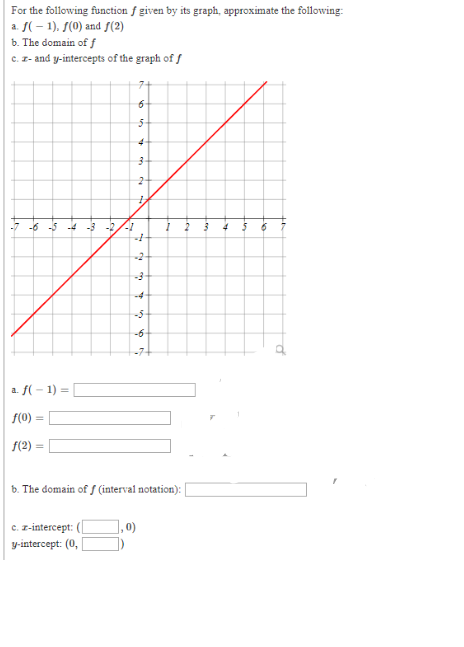For the following function f given by its graph, approximate the followingf(1) f(0) and f(2)ab. The domain of fc. - and y-intercepts of the graph of f7+65442--6 -5 -4-3 -252-3--4--6-7a. f1)=f(0) =f(2) =b. The domain of f (interval notation):], 0)c. z-intercept:y-intercept: (0,.

Questionhelp_outlineImage TranscriptioncloseFor the following function f given by its graph, approximate the following f(1) f(0) and f(2) a b. The domain of f c. - and y-intercepts of the graph of f 7+ 6 5 44 2- -6 -5 -4-3 -2 5 2 -3- -4- -6 -7 a. f1) = f(0) = f(2) = b. The domain of f (interval notation): ], 0) c. z-intercept: y-intercept: (0, . fullscreen
Step 1

a. (-1,0) is on the graph

This means f(-1)=0

(0,1) is on the graph, so f(0)=1

(2,3) is on the graph so, f(2)=3

Answer(a): f(-1)=0, f(0)=1, f(2)=3

Step 2

Domain is the x values which f(x) takes.

There x takes all values for x.

So, domai...

Want to see the full answer?

See Solution

Want to see this answer and more?

Our solutions are written by experts, many with advanced degrees, and available 24/7

See Solution
Tagged in

Algebra